Subsections

## Background

Maple has several commands for computing derivatives. The most common ones are the diff command for differentiating expressions and the D operator for differentiating expressions. These are the commands we will consider here. A related commmand that we will not consider here is the implicitdiff command for finding derivatives of expressions implicitly.

### Functions and Expressions in Maple

Before introducing the commands for differentiation, we need to review the concepts of expression and function in Maple. An expression is a collection of mathematical symbols, for example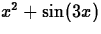and x2-y2 are both expressions. A function, on the other hand, is a rule for associating an output value with an input value. That is, a function defines a process for converting an input into an output.

The connection is that expressions are often used to define a function. That is, we could let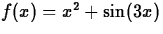, which defines a function f. The rule for this function is to substitute a value for x into the expressionto obtain the output value. Not all expressions can be used to define functions, however, and not all functions are defined by expressions so these are really distinct mathematical objects.

Maple mimics this mathematical distinction between expression and function. You can define expressions in Maple and even label them for later use with commands like the one below.

  > p := x^2+sin(3*x);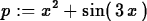This is an expression not a function, which means there is no rule associated with it. Thus evaluating the expression at a specific value of x requires the subs command, as in the following example.
  > subs(x=2,p);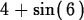The syntax for defining a function in Maple uses an arrow to make the idea of a function as a process explicit. For example, we can define a function f in Maple using the expressionwith the following command.
  > f := x -> x^2+sin(3*x);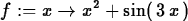Evaluating our function at a specific value of x is now easy.
  > f(2);One final thing to note is that Maple will use f to denote the function we have defined, but will use f(x) to denote the expression used to define the function.

### Something you should never do

Students often want to define a function with a command like the following.
  > F(x) := x^2+3;Since Maple doesn't complain, students often think that what they've done is correct. The output from the following commands, however, shows that the object we've defined doesn't behave like a function.
  > F(x);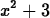> F(1);> F(t);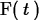> F(2*x);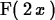What is happening here is that we've defined something called a table in Maple. For the input x it gives the output x2+3, but it doesn't behave like a function and doesn't give the value we wanted for any other input.

### The Maple D and diff commands

These commands can be summarized simply as follows.
• The D operator acts on a function to produce the derivative of that function.
• The diff command acts on an expression and differentiates that expression with respect to a variable specified by the user.

When you use the D operator to compute the derivative of a function, the result is also a function, as shown below.

  > D(f);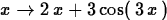If you provide a label, then you get a function you can use later in the session,
  > df := D(f);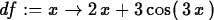However, this is usually not necessary. See the examples below.

If you want to evaluate the derivative at a specific value of x or just get the expression for the derivative, you can use the following forms of the D operator.

  > D(f)(2);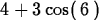> D(f)(x);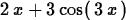This last form is the one to use for plotting, as shown below.
  > plot(D(f)(x),x=-2..2);


The D operator cannot be used on expressions, for example trying to use it to differentiate the expresssion we defined above results in an error.

  > D(p);

Error, (in D) univariate operand expected

If you recall that Maple uses f(x) to refer to the expresssion that is used to define f, then the following error shouldn't surprise you.
  > D(f(x));

Error, (in D) univariate operand expected


To differentiate expressions, you need to use the diff command. Here is an example.

  > diff(p,x);The diff command can also be applied to functions as shown below.
  > diff(f(x),x);Note, however, that the result of the diff command is an expression, not a function. This means that computing the value of the derivative at a specific value of x requires you to use the subs command.

### Higher order derivatives

Computing higher order derivatives with the diff command is fairly simple. The following commands compute the second, third and fourth derivatives of our function f.
  > diff(f(x),x,x);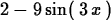> diff(f(x),x,x,x);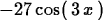> diff(f(x),x,x,x,x);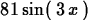The D operator can also be used to compute higher order derivatives. One way to compute the second derivative is shown below.

  > D(D(f));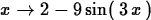This get cumbersome if you need derivatives of higher order, but there is another way to do it. The examples below show how to compute the second, third, and fourth derivatives using this alternate syntax.
  > D[1,1](f);> D[1,1,1](f);> D[1,1,1,1](f);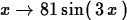There is an explanation for this syntax, but it probably won't make sense to you until you see partial derivatives in Calc IV.

### Tangent Lines

In this subsection, we compute the tangent line at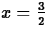to our function f using first the D operator and then the diff command. This gives one example where the D operator is probably easier to use than the diff command.

To find the tangent line at atto our function f, we need two pieces of information. One is the slope of the tangent line, which is given by, and the other is a point on the line, which is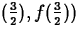. Then, using the point-slope formula, the equation for the tangent line isImplementing this using the D operator is relatively simple, as shown below.

  > tf := x -> D(f)(3/2)*(x-3/2)+f(3/2);> plot({f(x),tf(x)},x=0..3);


Using the diff command to compute the tangent line is a little harder, because the subs command must be used.

  > tf2  := x -> subs(x=3/2,diff(f(x),x))*(x-3/2)+f(3/2);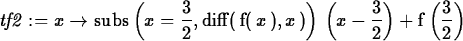> plot({f(x),tf2(x)},x=0..2);Next: Exercises Up: Derivatives Previous: Purpose

William W. Farr
11/18/1997